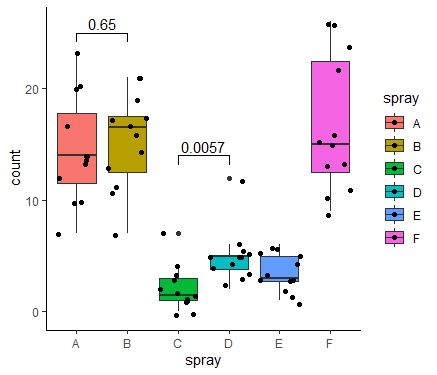# ggplot box plot plus p value

#### Introduction

When ggplot is making box plots or dot plots, it can actually check and add p-values, just use the functions of the ggpubr package

#### Code exmaple

``````library(ggplot2)
library(ggpubr)``````

Do t test for groups A, B, C, and D respectively, set in the comparisons parameter,the inspection method is set in the method, can be t.test, t.test, t.test, wilcox.test, wilcox.test, wilcox.test, anova, anova, kruskal.test, kruskal.test``````ggplot(InsectSprays, aes(x = spray, y= count,fill = spray)) + geom_boxplot() + theme_classic()+
geom_point(position = "jitter") +
stat_compare_means(method = "t.test",
comparisons = list(c("A", "B"),c("C","D")),
label.y = c(25, 14)
)
``````

Select only groups A and B, use label.x.npc = .5, set the label in the middle``````dp <- InsectSprays[InsectSprays\$spray %in% c("A", "B"),]
dp\$spray <- as.factor(dp\$spray)
ggplot(dp, aes(x = spray, y= count,fill = spray)) + geom_boxplot() + theme_classic()+
geom_point(position = "jitter") +
stat_compare_means(label.x.npc = .5)``````